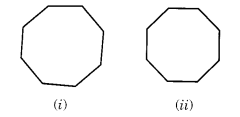Understanding Elementary Shapes (Exercise – 5.8)Ex 5.8 Question 1.

Examine whether the following are polygons. If anyone among them is not, say why?Solution:
(a) As the given figure is not closed. Therefore, it is not a polygon.
(b) The given figure is a polygon. It is made of six sides.
(c) The given figure is not a polygon because it is not made by line segments.
(d) The given figure is not a polygon because it is not made by line segments it has a curved surface also.

Ex 5.8 Question 2.

Name the polygon.Make two more examples of each of these.
Solution:(b) Triangle Examples:(c) Pentagon Examples:(d) Octagon Examples:Ex 5.8 Question 3.

Draw a rough sketch of a regular hexagon. Connecting any three of its vertices, draw a triangle. Identify the type of the triangle you have drawn.
Solution:Ex 5.8 Question 4.

Draw a rough sketch of a regular octagon. (Using squared paper if you wish). Draw a rectangle by joining exactly four of the vertices of the octagon.
Solution:Ex 5.8 Question 5.

A diagonal is a line segment that joins any two vertices of the polygon and is not a side of the polygon. Draw a rough sketch of a pentagon and draw its diagonals.
Solution: